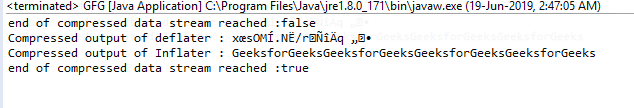Skip to content
Related Articles
Inflater finished() function in Java with examples
• Last Updated : 24 Jun, 2019

The finished() function of the Inflater class returns true if the end of compression data stream has been reached.

Function Signature:

```public boolean finished()
```

Syntax:

```i.finished();
```

Parameter: The function requires no parameter

Return Type: The function returns boolean value, returns true if all the input is uncompressed and stored in the given buffer, else return false.

Exception: The function does not throw any exception

Example 1: use of finished function

 `// Java program to describe the use``// of finished() function`` ` `import` `java.util.zip.*;``import` `java.io.UnsupportedEncodingException;`` ` `class` `GFG {``    ``public` `static` `void` `main(String args[])``        ``throws` `UnsupportedEncodingException,``               ``DataFormatException``    ``{`` ` `        ``// compress the data`` ` `        ``// deflater``        ``Deflater d = ``new` `Deflater();`` ` `        ``// get the text``        ``String pattern = ``"GeeksforGeeks"``, text = ``""``;`` ` `        ``// generate the text``        ``for` `(``int` `i = ``0``; i < ``4``; i++)``            ``text += pattern;`` ` `        ``// set the input for deflator``        ``d.setInput(text.getBytes(``"UTF-8"``));`` ` `        ``// finish``        ``d.finish();`` ` `        ``// output bytes``        ``byte` `output[] = ``new` `byte``[``1024``];`` ` `        ``// compress the data``        ``int` `size = d.deflate(output);`` ` `        ``// end``        ``d.end();`` ` `        ``// end of compression`` ` `        ``// use Inflater to get back the original data`` ` `        ``// Inflater``        ``Inflater i = ``new` `Inflater();`` ` `        ``// value returned by finished function``        ``System.out.println(``"end of compressed data stream reached :"``                           ``+ i.finished());`` ` `        ``// set the input for inflator``        ``i.setInput(output);`` ` `        ``// output bytes``        ``byte` `inflater_output[] = ``new` `byte``[``1024``];`` ` `        ``// uncompress the data``        ``int` `org_size = i.inflate(inflater_output);`` ` `        ``// output of inflater and deflater``        ``System.out.println(``"Compressed output of deflater : "``                           ``+ ``new` `String(output));``        ``System.out.println(``"Compressed output of Inflater : "``                           ``+ ``new` `String(inflater_output, ``"UTF-8"``));`` ` `        ``// value returned by finished function``        ``System.out.println(``"end of compressed data stream reached :"``                           ``+ i.finished());`` ` `        ``// end``        ``i.end();``    ``}``}`

Output:Attention reader! Don’t stop learning now. Get hold of all the important Java Foundation and Collections concepts with the Fundamentals of Java and Java Collections Course at a student-friendly price and become industry ready. To complete your preparation from learning a language to DS Algo and many more,  please refer Complete Interview Preparation Course.

My Personal Notes arrow_drop_up